# Exponential sum estimates

Exponential sums have the form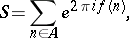whereis a finite set of integers andis a real-valued function (cf. also Trigonometric sum). The basic problem is to show, under suitable circumstances, that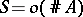as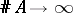. Unless there are obvious reasons to the contrary one actually expectsto have order around. Exponential sums in more than one variable also occur, and much of what is stated below can be generalized to such sums.

## Arithmetic sums.

There are two common types of exponential sum encountered in analytic number theory. In the first type, one starts with polynomials, a positive integer modulus, and a finite interval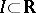. One then takesas the set of integers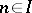for which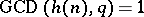, and sets, where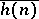is any integer for which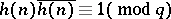. When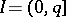, such a sum is called complete. Whenone may estimate the incomplete sum in terms of complete ones via the bound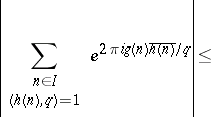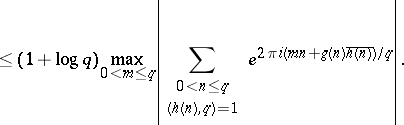Complete sums have multiplicative properties which enable one to reduce consideration to the case in whichis a prime number or a power of a prime number. Moreover, Weil's Riemann hypothesis for curves over finite fields (see [a4], for example) shows that, ifis prime, thenexcept when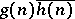is constant modulo. For powers of a prime number the situation is more complicated, but broadly similar. It follows that, for fixedandandprime, the incomplete sum will beas soon as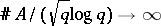. It is an important outstanding problem (as of 2000) to improve on this in general.

## Analytic sums.

The second type of exponential sum arises whenextends to a suitably smooth function on a real interval. Important examples correspond tofor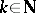, or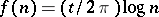, which occur in the theory of Waring's problem and the Riemann zeta-function, respectively (cf. also Waring problem; Zeta-function). Let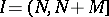with positive integers. One typically imposes the condition that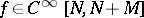with(a1)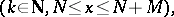for suitable constants,. Methods due to H. Weyl (see [a5], Chap. 2), J. van der Corput (see [a1]), I.M. Vinogradov and N.M. Korobov (see [a3], Chap. 6), and E. Bombieri and H. Iwaniec (see [a2]) have been used for sums of this type.

### Van der Corput's method.

Of the above approaches, the method of van der Corput is perhaps the most versatile. It is based on two processes, which convert the original suminto other sums. The A-process uses the inequality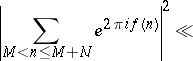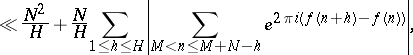wheresatisfies. This has the effect of replacingby a functionwhich satisfies (a1) with a smaller value of. To describe the B-process it is assumed thatis decreasing, and one writes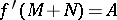,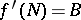and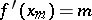for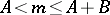. The B-process then derives from the the estimate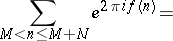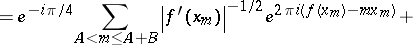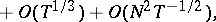which may be viewed as a form of the Poisson summation formula. When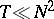, the B-process transformsinto a sum with the same value ofbut a shorter range. In applications one uses the A-process repeatedly, with various values of, until one has a sum to which the B-process can be applied effectively. One may then estimate the resulting shorter sum either via a trivial bound or by repeating the argument. In particular,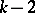iterations of the A-process, followed by the B-process and a trivial estimate lead to van der Corput'sth derivative estimate: Let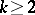be an integer, and letwith,integers. Suppose that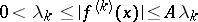on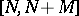. Then, if, one has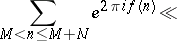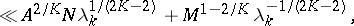uniformly in.

### Exponent pairs.

Many bounds of the form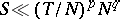subject to (a1), or similar slightly stronger conditions, have been proved. If one has such a bound, one says that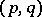is an exponent pair. Thus, the van der Corput third-derivative estimate shows thatis an exponent pair. The case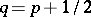is of particular interest, since it leads to a bound for the Riemann zeta-function, of the form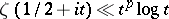for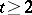. Although van der Corput's method leads to a rich source of exponent pairs, better results can be derived by more complicated methods. Thus, M.N. Huxley [a2], Chap. 17, shows thatis an exponent pair whenever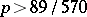, using the Bombieri–Iwaniec method. It is conjectured thatis an exponent pair for any positive. This can be seen as a generalization of the Lindelöf hypothesis for the Riemann zeta-function.

### The Vinogradov–Korobov method.

When (a1) holds withlarger than about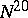, the van der Corput method is inferior to that given by Vinogradov and Korobov. This range of values is important in establishing zero-free regions for the Riemann zeta-function, for example. The method reduces the problem to an estimate for the number of solutions of the simultaneous equations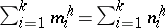for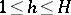with positive integer variables. This is provided by Vinogradov's mean value theorem (cf. also Vinogradov method).